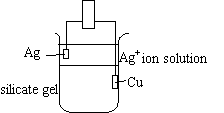## 20.5 Electrochemical Cells Using Sodium Silicate

Chemical Concepts Demonstrated: Galvanic and voltaic cells

Demonstration:

 The silicate gel is made up of sodium silicate dissolved in water and a Cu2+ solution made of Cu(NO3)2 * H2O dissolved in water with acetic acid. A copper metal electrode is inside the gel. A metal ion solution (Ag+, Zn2+, or Pb2+) is poured over the gel. A corresponding metal electrode is placed in the solution.Observations:

The gel allows the copper metal to act as a voltaic cell with the metal ion solution.

Explanation (including chemical equations):

The Ag +/Ag half-cell coupled with the Cu 2+ /Cu half-cell yields a standard-state cell potential of 0.46 V.

 anode: Cu (s) ---> Cu 2+ (aq) + 2 e- Eo = - 0.34 V cathode: Ag + (aq) + e- --->  Ag(s) Eo = 0.80 V Cu (s) + 2 Ag + (aq) ---> Cu 2+ (aq) + 2 Ag(s) Eo cell  =  0.46 V

If the Ag +/Ag half-cell is replaced using the Pb 2+/Pb or the Zn 2+/ Zn half reactions, the leads to the voltmeter would need to be reversed in order to set up a volaic cell:

 anode: Cu (s) ---> Cu 2+ (aq) + 2 e- Eo = - 0.34 V cathode: Pb 2+ (aq) +  2 e- --->  Pb (s) Eo =  - 0.13 V Cu (s) + Pb 2+ (aq) --->  Cu 2+ (aq) + Pb (s) Eo cell  =  -0.47 V

or:

 anode: Cu (s) ---> Cu 2+ (aq) + 2 e- Eo =  - 0.34 V cathode: Zn 2+ (aq) + 2 e- ---> Zn (s) Eo =  - 0.76 V Cu (s) + Zn 2+ (aq) ---> Cu 2+ (aq) + Zn (s) Eo cell  = -1.10 V